# What Makes 689 Amazing?

689 is an amazing number for several reasons. I decided to make graphics to illustrate many of those ways. (689’s factoring information is at the end of this post.)

689 is the sum of consecutive prime numbers 227, 229, and 233.

Also 689 is the sum of the primes from 83 to 109. Do you know what those 7 prime numbers are?

Stetson.edu informs us that 689 is the smallest number that can be expressed as the sum of three different square numbers NINE ways. I decided to figure out what those nine ways are and make this first graphic to share with you:Note: 614 can also be expressed as the sum of 3 squares 9 different ways, but one of those ways is 17² + 17² + 6² = 614, and that duplicates 17² in the same sum.

689 is the same number when it is turned upside down. Numbers with that characteristic are called Strobogrammatic numbers.689 BASE 10 isn’t a palindrome, but 373 BASE 14 is; note that 3(196) + 7(14) + 3(1) = 689

Both of 689’s prime factors have a remainder of 1 when divided by 4, so they are hypotenuses of Pythagorean triples. That fact also means 689 can be expressed as the sum of two square numbers TWO different ways, and it makes 689 the hypotenuse of FOUR Pythagorean triples.  Can you tell by looking at the graphic which two are primitive and which two aren’t?689 is the sum of consecutive numbers three different ways:

• 344 + 345 = 689; that’s 2 consecutive numbers.
• 47 + 48 + 49 + 50 + 51 + 52 + 53 + 54 + 55 + 56 + 57 + 58 + 59 = 689; that’s 13 consecutive numbers.
• 14 + 15 + 16 + 17 + 18 + 19 + 20 + 21 + 22 + 23 + 24 + 25 + 26 + 27 + 28 + 29 + 30 + 31 + 32 + 33 + 34 + 35 + 36 + 37 + 38 + 39 = 689; that’s 26 consecutive numbers.

Now you have a few reasons why 689 is an amazing number. 13 and 53 were part of some of those reasons so it shouldn’t surprise anyone to see 13 and 53 pop up in its factoring information, too:

——————————————————————————

• 689 is a composite number.
• Prime factorization: 689 = 13 x 53
• The exponents in the prime factorization are 1 and 1. Adding one to each and multiplying we get (1 + 1)(1 + 1) = 2 x 2 = 4. Therefore 689 has exactly 4 factors.
• Factors of 689: 1, 13, 53, 689
• Factor pairs: 689 = 1 x 689 or 13 x 53
• 689 has no square factors that allow its square root to be simplified. √689 ≈ 26.248809.# 685 Is the Sum of Two Squares Two Different Ways

Because both of its prime factors are hypotenuses of primitive Pythagorean triples, 685 is the hypotenuse of FOUR Pythagorean triples. Two are primitive; two are not:

• 37-684-685 which was calculated from 19² – 18², 2(19)(18), 19² + 18²
• 156-667-685 which was calculated from 2(26)(3), 26² – 3², 26² + 3²
• 411-548-685 (What factor of 685 is the greatest common factor of those 3 numbers?)
• 440-525-685 (and what is their greatest common factor?)

As you may have notice from those calculations, 685 is the sum of two squares two different ways:

• 19² + 18² = 685
• 26² + 3² = 685

685 is the 19th centered square number because 18 and 19 are consecutive numbers and 19² + 18² = 685. There are 685 small squares of various colors in this graphic.—————————————————————————————————

• 685 is a composite number.
• Prime factorization: 685 = 5 x 137
• The exponents in the prime factorization are 1 and 1. Adding one to each and multiplying we get (1 + 1)(1 + 1) = 2 x 2 = 4. Therefore 685 has exactly 4 factors.
• Factors of 685: 1, 5, 137, 685
• Factor pairs: 685 = 1 x 685 or 5 x 137
• 685 has no square factors that allow its square root to be simplified. √685 ≈ 26.172505.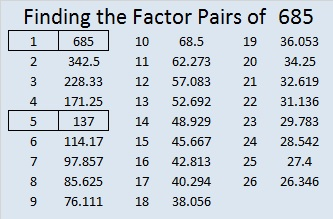—————————————————————————————————

# 674 and Level 1

674 is the hypotenuse of the Pythagorean triple 350-576-674. What is the greatest common factor of those three numbers?

674 is a leg in exactly one Pythagorean triple: 674-113568-113570, and those three numbers have the exact same greatest common factor as the triple above.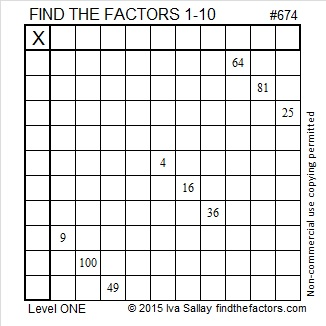Print the puzzles or type the solution on this excel file: 10 Factors 2015-11-09

—————————————————————————————————

• 674 is a composite number.
• Prime factorization: 674 = 2 x 337
• The exponents in the prime factorization are 1 and 1. Adding one to each and multiplying we get (1 + 1)(1 + 1) = 2 x 2 = 4. Therefore 674 has exactly 4 factors.
• Factors of 674: 1, 2, 337, 674
• Factor pairs: 674 = 1 x 674 or 2 x 337
• 674 has no square factors that allow its square root to be simplified. √674 ≈ 25.96151.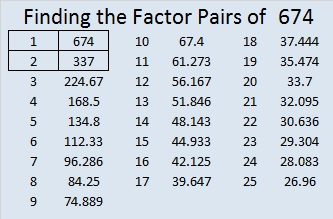—————————————————————————————————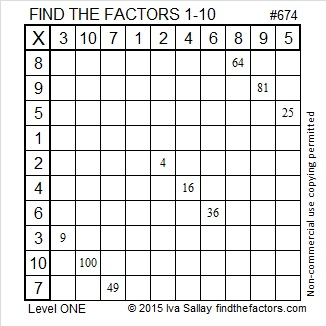# 663 Peanut Butter Kiss Puzzle

It’s easy to see that 663 is divisible by three.

That may not be very interesting at all, but…..

Because 13 and 17, 663’s other two prime factors, have a remainder of one when each is divided by four, 663 is the hypotenuse of FOUR Pythagorean triples. Can you find the greatest common factor of each triple?

• 420-513-663
• 63-660-663
• 255-612-663
• 312-585-663

—————————————————————————————————

Art is not one of my strongest skills, but here is my attempt to make a puzzle that looks like a peanut butter kiss, a traditional Halloween candy:Color can be inviting, but it can also be distracting. Here is the same puzzle without the added color:Print the puzzles or type the solution on this excel file: 10 Factors 2015-10-26

—————————————————————————————————

• 663 is a composite number.
• Prime factorization: 663 = 3 x 13 x 17
• The exponents in the prime factorization are 1, 1, and 1. Adding one to each and multiplying we get (1 + 1)(1 + 1)(1 + 1) = 2 x 2 x 2 = 8. Therefore 663 has exactly 8 factors.
• Factors of 663: 1, 3, 13, 17, 39, 51, 221, 663
• Factor pairs: 663 = 1 x 663, 3 x 221, 13 x 51, or 17 x 39
• 663 has no square factors that allow its square root to be simplified. √663 ≈ 25.748786.—————————————————————————————————Thank you for sharing the solution, Ricardo, and happy Halloween:

# 629 and Level 1

629 is the sum of the 17 prime numbers from 7 to 71. Both of its prime factors, 17 and 37, are included in that list.

17 and 37 are both 10 numbers away from their average, 27. That means that 629 + 10² = 729 or 27².

25² + 2² = 629 and 23² + 10² = 629. Notice that 629 plus or minus 100 is a square number.

Both of 629’s prime factors have a remainder of one when divided by four so 629 is the hypotenuse of four Pythagorean triples, two of which are primitives.

• 100-621-629, a primitive that reminds me of another primitive, 20-21-29
• 204-595-629, three numbers whose greatest common factor is 17
• 296-555-629, three numbers whose greatest common factor is 37
• 429-460-629, a primitive whose shorter leg is exactly 200 less than its hypotenuse.Print the puzzles or type the solution on this excel file: 10 Factors 2015-09-28

—————————————————————————————————

• 629 is a composite number.
• Prime factorization: 629 = 17 x 37
• The exponents in the prime factorization are 1 and 1. Adding one to each and multiplying we get (1 + 1)(1 + 1) = 2 x 2 = 4. Therefore 629 has exactly 4 factors.
• Factors of 629: 1, 17, 37, 629
• Factor pairs: 629 = 1 x 629 or 17 x 37
• 629 has no square factors that allow its square root to be simplified. √629 ≈ 25.079872.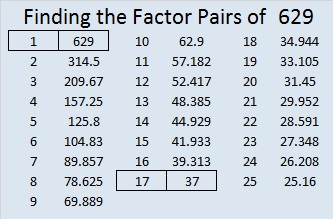—————————————————————————————————# 595 and Level 3

595 = (34/2)(34 + 1) so 595 is the 34th triangular number.

Thus 1 + 2 + 3 + 4 + . . . . . + 31 + 32 + 33 + 34 = 595

595 is also the hypotenuse of four Pythagorean triples. Each of these triples has its own greatest common factor.

• 91-588-595
• 252-539-595
• 280-525-595
• 357-476-595Print the puzzles or type the solution on this excel file: 12 Factors 2015-08-24

—————————————————————————————————

• 595 is a composite number.
• Prime factorization: 595 = 5 x 7 x 17
• The exponents in the prime factorization are 1, 1, and 1. Adding one to each and multiplying we get (1 + 1)(1 + 1)(1 + 1) = 2 x 2 x 2 = 8. Therefore 595 has exactly 8 factors.
• Factors of 595: 1, 5, 7, 17, 35, 85, 119, 595
• Factor pairs: 595 = 1 x 595, 5 x 119, 7 x 85, or 17 x 35
• 595 has no square factors that allow its square root to be simplified. √595 ≈ 24.39262.—————————————————————————————————

A Logical Approach to solve a FIND THE FACTORS puzzle: Find the column or row with two clues and find their common factor. Write the corresponding factors in the factor column (1st column) and factor row (top row).  Because this is a level three puzzle, you have now written a factor at the top of the factor column. Continue to work from the top of the factor column to the bottom, finding factors and filling in the factor column and the factor row one cell at a time as you go.# 565 and Level 1

565 is the sum of consecutive primes: 181 + 191 + 193 = 565.

565 is the sum of two squares two different ways: 565 = (22^2) + (9^2), and 565 = (23^2) + (6^2).

565 is the hypotenuse of four Pythagorean triples. The greatest common factor of two of them is 1 because they are primitives. Which of these triples are not primitive, and what is the greatest common factor of each of them?

• 75-560-565
• 276-493-565
• 339-452-565
• 396-403-565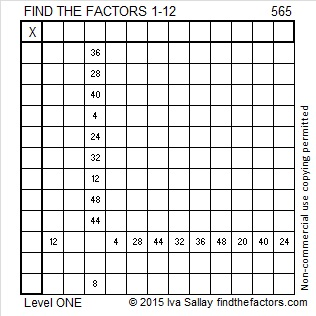Print the puzzles or type the solution on this excel file: 12 Factors 2015-07-27

—————————————————————————————————

• 565 is a composite number.
• Prime factorization: 565 = 5 x 113
• The exponents in the prime factorization are 1 and 1. Adding one to each and multiplying we get (1 + 1)(1 + 1) = 2 x 2 = 4. Therefore 565 has exactly 4 factors.
• Factors of 565: 1, 5, 113, 565
• Factor pairs: 565 = 1 x 565 or 5 x 113
• 565 has no square factors that allow its square root to be simplified. √565 ≈ 23.7697—————————————————————————————————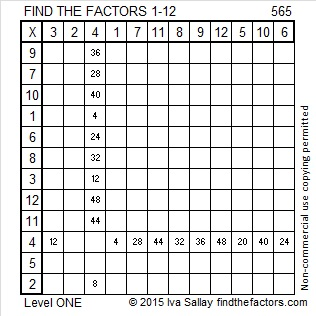# 545 and Level 2

The first few centered square numbers are 1, 5, 13, 25, 41, and 61. Starting in the center of this multi-colored square, can you locate each of those centered square numbers? 545 is the 17th centered square number. This wikipedia link explains the relationship between centered square numbers and the more familiar square numbers like 1, 4, 9, 16, 25 and 36. It also explains that every centered square number except 1 is the hypotenuse of a Pythagorean triple.If you have difficulty seeing those first few centered square numbers, perhaps this will help: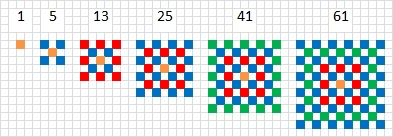545 is a centered square number because 16 and 17 are consecutive numbers and (16^2) + (17^2) = 545.

It is probably less exciting that (23^2) + (4^2) = 545.

545 is the hypotenuse of four Pythagorean triples. Which of these triples are primitives and which of them aren’t? The ones with greatest common factors greater than one are not primitive:

• 33-544-545
• 184-513-545
• 300-455-545
• 327-436-545Print the puzzles or type the solution on this excel file: 10 Factors 2015-07-06

—————————————————————————————————

• 545 is a composite number.
• Prime factorization: 545 = 5 x 109
• The exponents in the prime factorization are 1 and 1. Adding one to each and multiplying we get (1 + 1)(1 + 1) = 2 x 2 = 4. Therefore 545 has exactly 4 factors.
• Factors of 545: 1, 5, 109, 545
• Factor pairs: 545 = 1 x 545 or 5 x 109
• 545 has no square factors that allow its square root to be simplified. √545 ≈ 23.345235—————————————————————————————————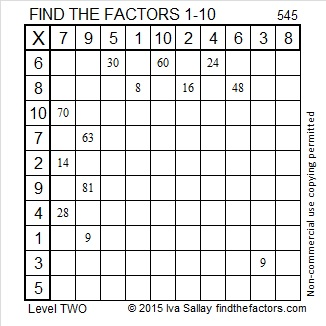# 520 and Level 5

520 is the hypotenuse of four Pythagorean triples. Can you find the greatest common factors of each of these triples:

• 128-504-520
• 200-480-520
• 264-448-520
• 312-416-520

520 = (23^2) – (3^2).Print the puzzles or type the solution on this excel file: 10 Factors 2015-06-08

—————————————————————————————————

• 520 is a composite number.
• Prime factorization: 520 = 2 x 2 x 2 x 5 x 13, which can be written 520 = (2^3) x 5 x 13
• The exponents in the prime factorization are 3, 1, and 1. Adding one to each and multiplying we get (3 + 1)(1 + 1)(1 + 1) = 4 x 2 x 2 = 16. Therefore 520 has exactly 16 factors.
• Factors of 520: 1, 2, 4, 5, 8, 10, 13, 20, 26, 40, 52, 65, 104, 130, 260, 520
• Factor pairs: 520 = 1 x 520, 2 x 260, 4 x 130, 5 x 104, 8 x 65, 10 x 52, 13 x 40, or 20 x 26
• Taking the factor pair with the largest square number factor, we get √520 = (√4)(√130) = 2√130 ≈ 22.8035085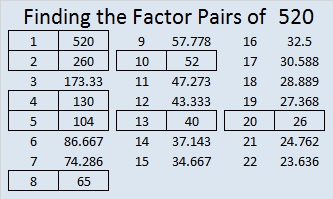—————————————————————————————————

# 485 and Level 5

485 is the hypotenuse of four Pythagorean triples. Which ones are primitive and which ones aren’t?

• 44-483-485
• 93-476-485
• 291-388-485
• 325-360-485Print the puzzles or type the solution on this excel file: 12 Factors 2015-05-04

—————————————————————————————————

• 485 is a composite number.
• Prime factorization: 485 = 5 x 97
• The exponents in the prime factorization are 1 and 1. Adding one to each and multiplying we get (1 + 1)(1 + 1) = 2 x 2 = 4. Therefore 485 has exactly 4 factors.
• Factors of 485: 1, 5, 97, 485
• Factor pairs: 485 = 1 x 485 or 5 x 97
• 485 has no square factors that allow its square root to be simplified. √485 ≈ 22.0227155—————————————————————————————————